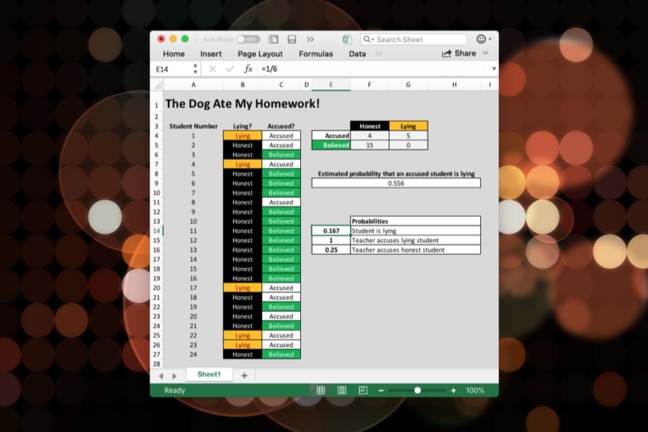## Want to keep learning?

This content is taken from the Cambridge University Press's online course, Teaching Probability. Join the course to learn more.
2.8

## Cambridge University PressWe can use a spreadsheet to simulate the results of the experiment

# Spreadsheet models for conditional probability

Download the supplied spreadsheet, ‘Simulation 2’. This shows how we can use a spreadsheet to simulate the results of ‘The dog ate my homework’.

Notice that the downloaded spreadsheet is set up with the probabilities incorporated into the spinners we used in the previous step - but these can be adjusted by changing the values in the range E14:E16.

Experiment with the spreadsheet. Use the Function key F9 to generate a new set of randomised results (if F9 does not work, typing a value into any blank cell and then moving to another cell should cause the spreadsheet to recalculate; and for users of Excel on Mac, hold down the Command key and press =).